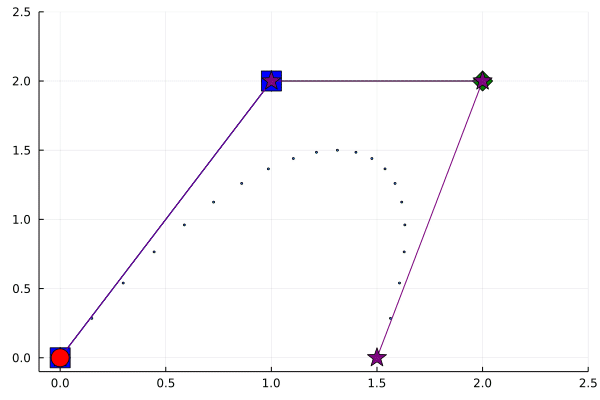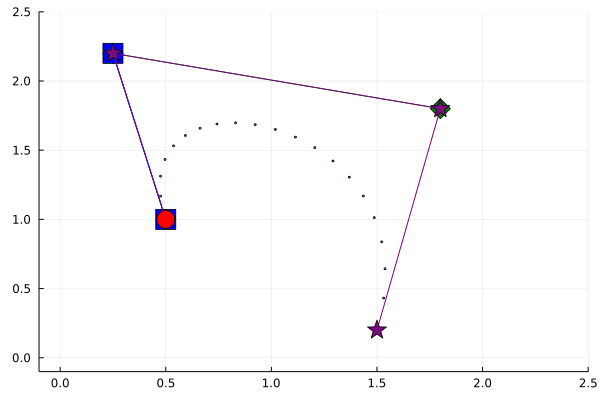I love small projects for helping me learn, especially programming. I’m still learning Julia, and have found myself wanting more “little silly things” I can digest and learn from. A lot of the projects I see in Julia are big mathematical models, and I’m just not ready to dive that deep yet.

This series of tweets caught my eye, partly because of the cool animation, but also the bite-sized amount of information it was conveying - that interpolation in Julia can be specified so easily, thanks in large part to the multiple dispatch design of the language.

“Surely I could get those 7 lines of code to run” I thought.

Entering the code into VScode was straightforward enough, no problems there. I could define the interpolation function

``````interpolate(a, b) = t -> ((1.0-t)*a + t*b)
``````

however extending the `*` and `+` methods did require me to `import Base:*` and `import Base:+` which I think I knew but had forgotten.

``````+(f::Function, g::Function) = x -> f(x) + g(x)
*(t::Number, g::Function) = x -> t * g(x)
``````

Defining the secondary and tertiary interpolations, also straightforward

``````bz1(p1, p2) = interpolate(p1, p2)
bz2(p1, p2, p3) = interpolate(bz1(p1, p2), bz1(p2, p3))
bz3(p1, p2, p3, p4) = interpolate(bz2(p1, p2, p3), bz2(p2, p3, p4))
``````

Now the tricky part - evaluating some of these. I knew that `a` and `b` represent points, but how to do that here? They’re not single numbers, but coordinates. I tried a `Tuple` as `(1, 2)` but that doesn’t seem to work. I do need to remember that `interpolate` is itself a function of `t`, so that needs to be specified as well. If I try to interpolate halfway between two “points” with `Tuple`s

``````interpolate((0,1), (1,2))(0.5)
ERROR: MethodError: no method matching *(::Float64, ::Tuple{Int64,Int64})
Closest candidates are:
*(::Any, ::Any, ::Any, ::Any...) at operators.jl:538
*(::Float64, ::Float64) at float.jl:405
*(::AbstractFloat, ::Bool) at bool.jl:112
``````

Okay, how about `Array`s?

``````interpolate([0,1], [1,2])(0.5)
2-element Array{Float64,1}:
0.5
1.5
``````

Huzzah!

After that, it’s a matter of generating the points specified by

``````bz3(p1, p2, p3, p4)(t)(t)(t)
``````

for various values of `t`. I did that with a `map` and joined the results back into a single `Array`

``````dots = map(i -> bz3(p1, p2, p3, p4)(i)(i)(i),collect(0:0.1:1))
dots = hcat(dots...)
dots
2×11 Array{Float64,2}:
0.5  0.47535  0.5368  0.66245  …  1.36905  1.4872  1.53815  1.5
1.0  1.3124   1.5312  1.6588      1.3052   1.0128  0.6436   0.2
``````

That was, I’d say, a success.

Drunk with confidence, I wanted to try to reproduce the animation from the tweet, so I dug into the documentation. It didn’t seem too bad, and I think I’ve managed to reproduce it pretty well

``````anim = @animate for t in collect(vcat(0:0.01:1,1:-0.01:0))
a = bz3(p1, p2, p3, p4)(t)(t)(t);
b1 = bz2(p1, p2, p3)(t)(t);
b2 = bz2(p2, p3, p4)(t)(t);
c1 = bz1(p1, p2)(t);
c2 = bz1(p2, p3)(t);
c3 = bz1(p3, p4)(t);
stars = hcat(p1, p2, p3, p4);
diamond1 = hcat(c1, c2);
diamond2 = hcat(c2, c3);
square = hcat(b1, b2);
plot(xlim = (-0.1,2.5), ylim = (-0.1,2.5), legend = false)
scatter!(dots[1,:], dots[2,:], markersize = 2)
plot!(diamond1[1,:], diamond1[2,:], markersize = 10, markershape = :diamond, color = :green)
plot!(diamond2[1,:], diamond2[2,:], markersize = 10, markershape = :diamond, color = :green)
plot!(square[1,:], square[2,:], markersize = 10, markershape = :square, color = :blue)
plot!(stars[1,:], stars[2,:], markersize = 10, markershape = :star, color = :purple)
scatter!(Tuple(a), markersize = 10, markershape = :circle, markercolor = :red)
end

gif(anim, fps = 24)
``````Moving the points around, I get a new version all of my ownI’m very happy with how these turned out, and I’ve learned a lot! A gist of the code to make these is hosted here: https://gist.github.com/jonocarroll/27f9b57332424ea50ec2970e74d8e3b3

If there are better ways to do any of the steps (there surely are) please feel free to let me know!